# Insertion Sort in C#

## Insertion Sort in C# with Examples

In this article, I am going to discuss the Insertion Sort in C# with Examples. Please read our previous article where we discussed Merge Sort in C# with Examples. The Insertion sort is a simple sorting algorithm. It is used mainly when the number of elements is small. It can also be useful when the input element is almost sorted, only a few elements are misplaced in a big array.

##### The Insertion Sort Algorithm:

The Insertion Sort Algorithm maintains two sub-lists named sorted sub-list and unsorted sub-list. Through each iteration, the sorted sub-list increased and the unsorted sub-list keeps on decreasing. Elements from the unsorted list are picked and placed at the right position on the sorted list.

##### Pictorial Representation of Insertion Sort:

In Order to sort an array, Insertion sort iterate each element one by one and if any element is not in the correct position which means its previous value is not less than the current element then picked up that element and compare it with the elements which are left side (sorted list) and placed it at the appropriate place.

###### Unsorted Array: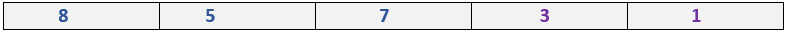###### Step1:

Compare 8 with 5 since 5 is smaller than 8 which means 5 is not in the correct position picked up element 5 and placed it in the 1st position and 8 to the 2nd position which basically means swap the values.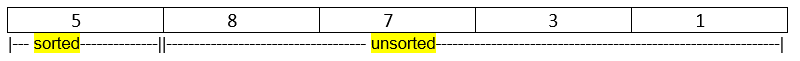###### Step2:

The 2nd step compares 7 with 8 and it’s previous all elements. Since 8 is greater than 7 which means 7 is not in the right position. So, our task is to find the correct position of 7 for that we need to compare all previous elements with 7. Because 7 is lesser than 8 and greater than 5 so the correct position of 7 will be in the 1st index (arr).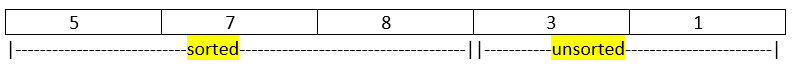###### Step3:

The 3rd step compares 3 with its previous element that is 8 since it is greater than 3 then continues the same process of comparing with its previous elements till we find the right position of 3.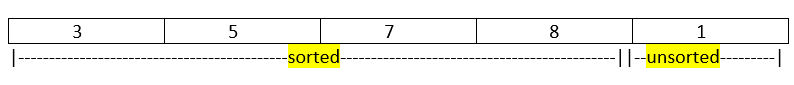###### Step4:

Now, we have only 1 element in our unsorted part which is 1 itself in this case. Again, the same rule needs to be followed to find the right position of 1 compare it with its previous elements till then the previous elements should be less than 1 and the successor elements should be greater than 1.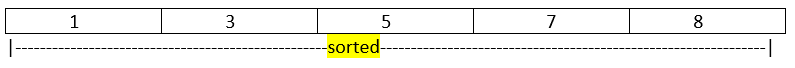So, finally after completing the steps, we have successfully sorted the array in ascending order. Congrats! You have learned the way how to sort an array element using the insertion sort algorithm.

Now, let’s go to the real fun which is the coding part. We are going to develop the C# program for insertion sort.

##### Program to implement insertion sort in C#
```using System;
namespace InsertionSortDemo
{
public class Example
{
public static void Main(string[] args)
{
int[] arr = new int { 8, 5, 7, 3, 1 };
int n = 5, i, j, val;
Console.WriteLine("Insertion Sort");
Console.Write("Initial array is: ");
for (i = 0; i < n; i++)
{
Console.Write(arr[i] + " ");
}
for (i = 1; i < n; i++)
{
val = arr[i];
for (j = i - 1; j >= 0;)
{
if (val < arr[j])
{
arr[j + 1] = arr[j];
j--;
arr[j + 1] = val;
}
else
{
break;
}
}
}
Console.Write("\nSorted Array is: ");
for (i = 0; i < n; i++)
{
Console.Write(arr[i] + " ");
}

}
}
}
```
###### Output: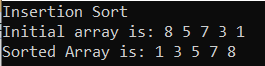##### Another way of implementing Insertion Sort in C#
```using System;
namespace InsertionSortDemo
{
public class Example
{
public static void Main(string[] args)
{
int[] arr = new int { 8, 5, 7, 3, 1 };
int n = 5, i, j, val;
Console.WriteLine("Insertion Sort");
Console.Write("Initial array is: ");
for (i = 0; i < n; i++)
{
Console.Write(arr[i] + " ");
}
for (i = 1; i < n; i++)
{
val = arr[i];
j = i - 1;
while (j >= 0 && arr[j] > val)
{
arr[j + 1] = arr[j];
j = j - 1;
}
arr[j + 1] = val;
}
Console.Write("\nSorted Array is: ");
for (i = 0; i < n; i++)
{
Console.Write(arr[i] + " ");
}

}
}
}
```
###### Output: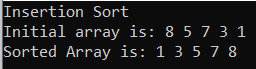##### Time Complexity of Insertion Sort

Insertion sort performs two operations: it scans through the list, comparing each pair of elements, and it swaps elements if they are out of order. Each operation contributes to the running time of the algorithm. If the input array is already in sorted order, insertion sort compares 0(n) elements and performs no swaps (the inner loop is never triggered).

Therefore, in the best case, insertion sort runs in 0(n) time. But in the worst and average-case time complexity will take 0(n2) time.

In the next article, I am going to discuss the Quick Sort in C# with examples. Here, in this article, I try to explain the Insertion Sort in C# with examples. I hope now you understood how Insertion Sort Algorithm Works in C# to sort an unsorted array.